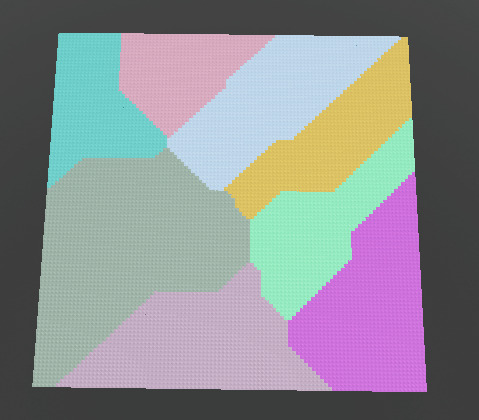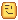# Voronoi Diagram Generator

So I made a Voronoi diagram in Roblox Studio using parts and a script obviously. I need help optimising it.

Script
``````SEED = workspace:WaitForChild("SEED").Value
X, Z = 100, 100

-- defines the folders for the parts
local one = Instance.new("Folder"); one.Name = "1"; one.Parent = workspace
local two = Instance.new("Folder"); two.Name = "2"; two.Parent = workspace
local three = Instance.new("Folder"); three.Name = "3"; three.Parent = workspace
local four = Instance.new("Folder"); four.Name = "4"; four.Parent = workspace

-- generates a grid of parts
local function genGrid(X, Z)
for z = 1, Z do
for x = 1, X do
local p = Instance.new("Part")
p.Size = Vector3.new(1.01, 1.01, 1.01)

p.Position = Vector3.new(x, 0, z)

p.Anchored = true
p.Parent = workspace

if p.Position.X <= X/2 and p.Position.Z <= Z/2 then
p.Parent = one
end

if p.Position.X <= X/2 and p.Position.Z >= Z/2 then
p.Parent = two
end

if p.Position.X >= X/2 and p.Position.Z <= Z/2 then
p.Parent = three
end

if p.Position.X >= X/2 and p.Position.Z >= Z/2 then
p.Parent = four
end

end

end
end

-- adds a random dot onto the grid (colors one of the parts black)
randomDots = {}
math.randomseed(SEED)
local function createRandomDot(folder)

local cube = math.random(1, #workspace:WaitForChild(folder):GetChildren())
local part

for i,v in ipairs(workspace:WaitForChild(folder):GetChildren()) do
if i == cube then
part = v
break
end
end

part.Color = Color3.fromRGB( math.random(55, 255), math.random(55, 255), math.random(55, 255) )

table.insert(randomDots, part)

end

-- expands area of biome
local function initVoronoi()

local newDots = {}

for i,v in next, randomDots do
for i2, v2 in pairs(v:GetTouchingParts()) do
if v2.Color == Color3.fromRGB(163, 162, 165) then
v2.Color = v.Color
table.insert(newDots, v2)
end
end
end

randomDots = newDots

end

genGrid(X, Z)

createRandomDot(1)
createRandomDot(2)
createRandomDot(3)
createRandomDot(4)

createRandomDot(1)
createRandomDot(2)
createRandomDot(3)
createRandomDot(4)

while wait() do
initVoronoi()
end
``````
What the script creates7 Likes

a for loop could be used here

EDIT: removed the first part, I’m an idiot

1 Like

hmm true, but would a for loop be better for performance?

honestly I’m not sure, but it is repeated code

I don’t check for loop performance much so maybe someone could test it

2 Likes

not sure if this matters but if it is at the top of a script it is technically already a global variable if you make it local

you shouldn’t use a single character for a variable name

1 Like

Even with the changes I tried I saw no difference between mine and your code in terms of performance, the only thing that could cause some slowdowns is your use of `wait()`, as it is unreliable and is bad usage unless you use larger numbers than the default, you could try using one of RunService’s events instead, but that could be its own issue as it would be tied to the server’s framerate, so I think you could try using this custom wait

Anyways, I went through your code as saw things that would definitely help make it more shorter and readable

My revised code
``````local SEED = workspace:WaitForChild("SEED").Value
local X, Z = 100, 100

-- defines the folders for the parts
local one = Instance.new("Folder"); one.Name = "1"; one.Parent = workspace
local two = Instance.new("Folder"); two.Name = "2"; two.Parent = workspace
local three = Instance.new("Folder"); three.Name = "3"; three.Parent = workspace
local four = Instance.new("Folder"); four.Name = "4"; four.Parent = workspace

local randomDots = {}
math.randomseed(SEED)

-- generates a grid of parts
local function genGrid(X, Z)
for z = 1, Z do
for x = 1, X do
local part = Instance.new("Part")
part.Size = Vector3.new(1.01, 1.01, 1.01)
part.Position = Vector3.new(x, 0, z)
part.Anchored = true

if part.Position.X <= X/2 then
part.Parent = part.Position.Z <= Z/2 and one or two
else
part.Parent = part.Position.Z <= Z/2 and three or four
end
end
end
end

-- adds a random dot onto the grid (colors one of the parts black)
local function createRandomDot(folder)
folder = tostring(folder)

local cube = math.random(#workspace[folder]:GetChildren())
local part

for index, child in ipairs(workspace[folder]:GetChildren()) do
if index ~= cube then
continue
end
part = child
break
end

part.Color = Color3.fromRGB(math.random(55, 255), math.random(55, 255), math.random(55, 255))
table.insert(randomDots, part)
end

-- expands area of biome
local function initVoronoi()
local newDots = {}

for _, dot in ipairs(randomDots) do
for _, touching in ipairs(dot:GetTouchingParts()) do
if touching.Color ~= Color3.fromRGB(163, 162, 165) then
continue
end
touching.Color = dot.Color
table.insert(newDots, touching)
end
end

randomDots = newDots
end

genGrid(X, Z)

for _ = 1, 2 do
for dot = 1, 4 do
createRandomDot(dot)
end
end

while true do
wait()
initVoronoi()
end
``````
My explanations
• It’s common nowadays to localized every variable you plan to use globally across your code, as it is slightlyyyyyyyyy (very unnoticable) faster, but generally it’s just used because everyone uses it so I made your variables local and to make it uniform with your functions, as you localize those

• I made your variable names more descriptive to help you and readers understand what they contain, example, I turned `v` in your `initVoronoi` function to `dot`, as it is in a `randomDots` table, `p` to `part` and so on. Being descriptive is the best thing you can do for yourself and others

• The way you set up which parent t he part belongs to is weird, no more than 1 condition is ever going to be met unless the position coincidentally exactly matches the half of one of the axis, which will make it go through all of them, which I’ve shortened using a combination of an if-else and a fake tenary,

``````local variable = condition and (condition true) or (condition false)
``````
• I removed your WaitForChilds as they’re not needed as everything you’re waiting for has already been made by the time the script runs

• I used a for loop to remove your code duplication for `createRandomDot`

• I removed the parameters for your `genGrid` function as the function can alreayd see what you’re passing in

*And some other things that don’t really need to be mentioned such as turning your `next` into an `ipairs` and making your `while wait() do` to a `while true do` with a `wait()` inside

Should hopefully still work exactly as how you intended

@ocula Thanks for mentioning you could for loop the numbers too, my 8pm brain forgot to notice that

1 Like

thank you so much for your revised code and your explanations. I truly appreciate it1 Like

As mentioned by @MightyPart, a for loop could improve the cleanliness of your code. It would have no difference in performance either. If anything, it’s a much cleaner way of organizing the way in which your code is structured.

So this:

``````createRandomDot(1)
createRandomDot(2)
createRandomDot(3)
createRandomDot(4)

createRandomDot(1)
createRandomDot(2)
createRandomDot(3)
createRandomDot(4)
``````

Would become this:

``````for _firstLoop = 1,2 do
for dot = 1,4 do
createRandomDot(dot)
end
end
``````

1 Like

if you wanted one for loop you could try this instead

``````for dot = 1, 8 do
createRandomDot(dot % 4 ~= 0 and dot % 4 or 4)
end
``````

the one @ocula gave and the one @EmbatTheHybrid gave are much cleaner but I thought I would say this

2 Likes

I believe that this generates a Manhattan Voronoi diagram. But how would you go about doing Euclidean Voronoi digaram with Delaunay triangulation?